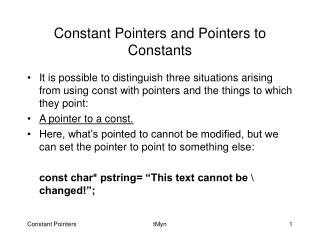# Constant Pointers and Pointers to Constants - PowerPoint PPT PresentationDownload PresentationConstant Pointers and Pointers to Constants

Constant Pointers and Pointers to ConstantsDownload Presentation## Constant Pointers and Pointers to Constants

- - - - - - - - - - - - - - - - - - - - - - - - - - - E N D - - - - - - - - - - - - - - - - - - - - - - - - - - -
##### Presentation Transcript

1. Constant Pointers and Pointers to Constants • It is possible to distinguish three situations arising from using const with pointers and the things to which they point: • A pointer to a const. • Here, what’s pointed to cannot be modified, but we can set the pointer to point to something else: const char* pstring= “This text cannot be \ changed!”; tMyn

2. #include "stdafx.h" #include <iostream> using namespace System; using namespace std; int main(array<System::String ^> ^args) { const int integer1=20; const int* pInt=&integer1; cout<<"Variable integer1 is a constant and cannot \ be changed.. " << endl<<"and it's value is now and always: " <<*pInt<<"."<<endl; const int integer2=40; pInt=&integer2; You couldn’t store the address of integer1 in a non-const pointer!! tMyn

3. cout<<"But it is still possible to set the pointer to \ point to something else!" <<endl<<"Variable integer2 is a constant and \ cannot be changed.. " << endl<<"and it's value is now and always: " <<*pInt<<"."<<endl; return 0; } tMyn

4. A constant pointer. • Here, the address stored in the pointer can’t be changed, so a pointer like this can only ever point to the address it is initialized with. • However, the contents of that address are not constant and can be changed. tMyn

5. #include "stdafx.h" #include <iostream> using namespace System; using namespace std; int main(array<System::String ^> ^args) { int integer1=20; int* const pInt=&integer1; cout<<"Pointer variable pInt is const, so it can \ only ever point to variable integer1." <<endl<<"However, the contents of variable integer1 is \ not const!"<<endl; The pointer pInt can only point to a non-const variable of type int! tMyn

6. integer1=30; cout<<"The value of the variable integer1 is this time " <<*pInt<<"."<<endl; return 0; } tMyn

7. A constant pointer to a constant. • Here, both the address stored in the pointer and the thing pointed to have been declared as constant, so neither can be changed. tMyn

8. #include "stdafx.h" #include <iostream> using namespace System; using namespace std; int main(array<System::String ^> ^args) { const int integer1=20; const int* const pInt=&integer1; cout<<"Pointer variable pInt is a constant \ pointer to a constant, "<<endl <<"so it is not possible to change \ what pInt points to."<<endl tMyn

9. <<"It is neither possible to change the value \ at the address it contains."<<endl <<"The value of the variable integer1 is now \ and always "<<*pInt<<"."<<endl; return 0; } tMyn

10. Naturally, this behaviour isn’t confined to the int types we have been dealing with so far. • This discussion applies to pointers of any type. tMyn